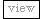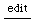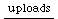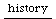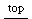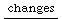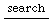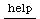## Using Patterns as Noise Source

Here is two ways to create a Noise Source from a Stream,
in this case using boolean Netwoks, Pboolnet by C.Ramacrishnan.
they are very inefficient, but can be seen as a sketch for whether it would be worthwhile
to implement them as C++ plugin.

```//for SC 3d5.1
//with CurveSeq
(
var n, l, net, pat;
n = 16; //number of levels
l = 32; //length of loop

net = {
Pboolnet(
Array.fill(l, {
[[0,1].choose, [\and, \or, \xor, \not].choose]
}).flat, inf
)
};
pat = 0;
n.do({ pat = pat + net.value });
pat = pat / 8 - 1; //scale down to -1/1

Library.put(\boolnet, pat);
Synth.scope(#{
var dt;
dt = MouseX.new([1/20, 1/1000]); //delta time
Pan2.ar(
CurveSeq.ar(1, Routine({
var stream;
stream = Library.at(\boolnet).asStream;
loop({
[dt.value, stream.next, \linear].yield;
});
})
), 0);
}, 0.1)
)

//with Sequencer
(
var n, l, net, pat;
n = 16; //number of levels
l = 32; //length of loop

net = {
Pboolnet(
Array.fill(l, {
[[0,1].choose, [\and, \or, \xor, \not].choose]
}).flat, inf
)
};
pat = 0;
n.do({ pat = pat + net.value });
pat = pat / 8 - 1; //scale down to -1/1

Library.put(\boolnet, pat);
Synth.scope(#{
var dt, out, stream;
out = Plug.ar(0, 0.0);
dt = Plug.kr(MouseX.kr([1/20, 1/1000])); //delta time
stream = Library.at(\boolnet).asStream;
Sequencer.ar({ out.line(stream.next, dt.poll); 0.0 }, Impulse.ar(dt.reciprocal));
Pan2.ar(out, 0);
}, 0.1)
)
```